Download Presentation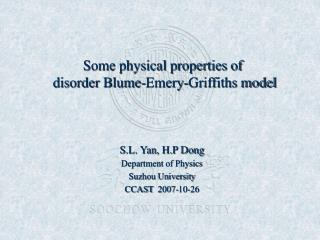Some physical properties of disorder Blume-Emery-Griffiths model

# Some physical properties of disorder Blume-Emery-Griffiths model - PowerPoint PPT Presentation

Some physical properties of disorder Blume-Emery-Griffiths model. S.L. Yan, H.P Dong Department of Physics Suzhou University CCAST 2007-10-26. Outlines. 1. Introduction 2. Theory 3. Results & discussions 4. Summary. 1. Introduction. Ising model and its developmentI am the owner, or an agent authorized to act on behalf of the owner, of the copyrighted work described.
Download Presentation## Some physical properties of disorder Blume-Emery-Griffiths model

An Image/Link below is provided (as is) to download presentation

Download Policy: Content on the Website is provided to you AS IS for your information and personal use and may not be sold / licensed / shared on other websites without getting consent from its author.While downloading, if for some reason you are not able to download a presentation, the publisher may have deleted the file from their server.

- - - - - - - - - - - - - - - - - - - - - - - - - - E N D - - - - - - - - - - - - - - - - - - - - - - - - - -
Presentation Transcript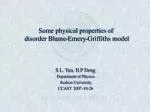### Some physical properties of disorder Blume-Emery-Griffiths model

S.L. Yan, H.P Dong

Department of Physics

Suzhou University

CCAST 2007-10-26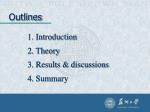Outlines

1. Introduction

2. Theory

3. Results & discussions

4. Summary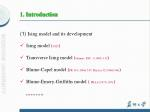1. Introduction

• Ising model and its development
• Ising model (1925)
• Transverse Ising model (Gennes, SSC, 1 (1963) 132)
• Blume-Capel model (PR 141 (1966) 517; Physica 32 (1966) 966)
• Blume-Emery-Griffiths model ( PRA 4 (1971) 1071)

………..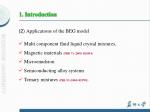1. Introduction

(2) Applications of the BEG model

• Multi component fluid liquid crystal mixtures,
• Magnetic materials (PRB 71 (2005) 024434)
• Microemulsion
• Semiconducting alloy systems
• Ternary mixtures (PRL 93 (2004) 025701)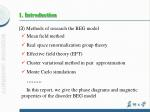1. Introduction

(3) Methods of research the BEG model

• Mean field method
• Real space renormalization group theory
• Effective field theory (EFT)
• Cluster variational method in pair approximation
• Monte Carlo simulations

………

In this report, we give the phase diagrams and magnetic properties of the disorder BEG model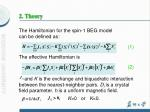2. Theory

The Hamiltonian for the spin-1 BEG model can be defined as:

(1)

The effective Hamiltonian is

(2)

and K is the exchange and biquadratic interaction between the nearest-neighbor pairs. Di is a crystal field parameter. h is a uniform magnetic field.

K>0 or K<0.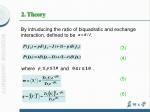2. Theory

By intruducing the ratio of biquadratic and exchange interaction, defined to be .

(3)

(4)

where

and

.

(5)

(6)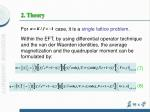2. Theory

For

case, it is a single lattice problem.

Within the EFT, by using differential operator technique and the van der Waerden identities, the average magnetization and the quadrupolar moment can be formulated by:

(7)

(8)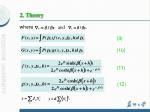2. Theory

where

and

(9)

(10)

(11)

(12)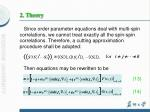2. Theory

Since order parameter equations deal with multi-spin correlations, we cannot treat exactly all the spin-spin correlations. Therefore, a cutting approximation procedure shall be adopted:

for

Then equations may be rewritten to be

(13)

(14)2. Theory

where z is the coordination number. By solving above-mention equations, the expression can be obtained:

(15)

According to Laudau theory, the second-order phase transition equation is determined by:

(16)

(17)2. Theory

where and are given by:

(18)

(19)2. Theory

The tricritical point at which the second-order phase

transition changes to the first-order one must satisfy

and

The first-order phase transition equation is determined

by

and2. Theory

For case, it is necessary to adopttwo sublattices. We can discuss staggered quadrupolar (SQ) phase and bicritical point of the BEG model. The Hamiltonian is

(1’)

(2’)

(3’)

(4’)

(5’)2. Theory

where

and

conditions.

The SQ phase satisfies

and

(6’)

(7’)

This is condition of the two-cycle fixed points. In addition, the paramagnetic phase corresponds

and

conditions.2. Theory

Thus, one has:

(8’)

Obviously, the paramagnetic phase satisfies the single fixed point condition. In order to obtain the SQ and the paramagnetic phase boundary, the single fixed point must bifurcate into two-cycle fixed points. The limit of stability for bifurcation of the fixed point is as follows:

(9’)2. Theory

The solution of the SQ-P phase boundary is given by equations (8’) and (9’).

The F-P second-order phase boundary is still given by equations (16) and (17).

The intersection of the SQ-P line and the F-P one is a BCP. The coordinate of the BCP is solution of the set of coupled equations (8’)-(9’) and (16)-(17).

For simplification, a simple cubic lattice is selected as the three-dimensional version3. Results & Discussions

(1) Critical behaviors of the BEG model for

• Ferromgnetic region;
• Reentrant phenomenon;
• Finite critical temperature;
• Double TCPs problem3. Results & Discussions

Shrink of Double TCPs3. Results & Discussions

Fig. (a) apparent reentrant phenomenon, Fig.(b) weak reentrant phenomenon

The TCP is depressed monotonically with decreasing crystal field concentration.3. Results & Discussions

• The bond percolation threshold is
• for
• The bond percolation threshold has many different values for
• There exist two double bond percolation threshold in Fig. 4(a)3. Results & Discussions

(2) Staggered quadrupolar phase and bicritical point

• SQ phase, SQ-P boundary, F-P boundary bicritical point
• different effect of two dilution factors

PRB 69 (2004) 0644233. Results & Discussions

Influence of different parameters on the SQ phase and the BCP3. Results & Discussions

(3) Magnetic properties of the BEG model for

Influence of positive and negative ratio onInitial magnetizations3. Results & Discussions

Influence of ratio and bond dilution on Initial magnetizations3. Results & Discussions

Influence of positive and negative ratio on susceptibility3. Results & Discussions

Influence of ratio and bond dilution on susceptibility3. Results & Discussions

The temperature dependence of the magnetization under different parameters3. Results & Discussions

(PRB 71 (2005) 024434)

Our result4. Summary

• Phase diagrams for positive ratio are compared with those for negative one. There exist double TCPs. The first-order phase transition is enlarged with increasing of ratio at a fixed random crystal field concentration, while the first-order phase transition will shrink due to a fixed bond dilution concentration. Bond percolation threshold is always p=0.2929 for and has many different values. The reentrant phenomenon is shown.
• The system has shown the SQ phase, the ferromagnetic phase and the BCP. A large negative ratio and two different dilution factors magnify the range of the SQ phase and reduce that of in or plane. These parameters can assist the reentrant behavior of the SQ-P lines and suppress that of the F-P lines. The influence of bond dilution on the BCP is dissimilar to that of anisotropy dilution.
• The initial magnetization curves and susceptibility curves exhibit an irregular behavior in the region of low temperature when the crystal field takes a smaller value. The peak of the susceptibility curve has a explicit decline. The magnetization curves transform from ferromagnetism to paramagnetism at high temperatures. The magnetization curves have a remarkable fluctuation process for a negative ratio and show a discontinuity due to larger crystal field and a positive ratio.